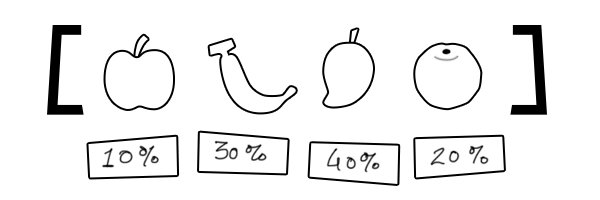# Posts

### UIE - Universal Information Extraction

UIE - a unified text-to-structure generation framework, which can universally model different IE tasks, adaptively generate targeted structures, and unfiedly learn general IE abilities from different knowledge sources.

### Vector Search Engine

Vector search engines or vector databases are a core piece of infrastracture that fuels every big deep learning deployment in industry.

### Language Model Is All You Need — Explores NLU as MRC QA

One Amazon Alexa AI’s new paper Language Model Is All You Need explores NLU problem as a QA problem. The original paper is on arXiv.

### ⛵ Learning Meaning in Natural Language Processing — The Semantics Mega-Thread

I found a very worthwhile article while surfing medium.com days ago. The article is a summary of a twitter thread which talked about meaning, semantics, language models, learning Thai and Java, entailment, co-reference — all in one fascinating thread. The original article is here.

### Filtered-Space Saving Top-K

Filtered-Space Saving (FSS) is a data structure and algorithm combination useful for accurately estimating the top k most frequent values appearing in a stream while using a constant, minimal memory footprint.

### Gödel’s First Incompleteness Theorem for Programmers

Gödel’s incompleteness theorems are two theorems of mathematical logic that demonstrate the inherent limitations of every formal axiomatic system containing basic arithmetic. These results, published by Kurt Gödel in 1931, are important both in mathematical logic and in the philosophy of mathematics.### Weighted Random: algorithms for sampling from discrete probability distributions

The optimal solution for weighted random should be the Alias Method. It requires $O(n)$ time to initialize, $O(1)$ time to make a selection, and $O(n)$ memory.

### Illustration of the logistic map

A Python implementation for illustrating the behavior of logistic map.